## Indicators

•Cited by SciELO
•Access statistics

•Cited by Google
•Similars in SciELO
•Similars in Google

## Print version ISSN 0120-5609

### Ing. Investig. vol.35 no.1 Bogotá Jan.Apr. 2015

#### http://dx.doi.org/10.15446/ing.investig.v35n1.45213

Estimation of the particle size distribution of colloids from multiangle dynamic light scattering measurements with particle swarm optimization

Estimación de distribución de tamaños de partículas de coloides a partir de mediciones de luz dinámica a múltiples ángulos con optimización por enjambre de partículas

L. A. Bermeo1, E. Caicedo2, L. Clementi3 and J. Vega4

1Leonardo A. Bermeo Varón. Electronic Engineer, M. Sc., Univ. del Valle, Colombia. Affiliation: D. Sc. Candidate, Federal Univ. of Rio de Janeiro, Brazil. E-mail: lebermeo@ufrj.br

2Eduardo Caicedo. Electric Engineer, Univ. del Valle, Colombia. M. Sc., Ph.D., Univ. Politécnica de Madrid, España. Senior Researcher, COLCIENCIAS, Colombia. Affiliation: Univ. del Valle, Percepción y Sistemas Inteligentes, PSI Group, Colombia. E-mail: eduardo.caicedo@correounivalle.edu.co

3Luis Clementi. Bioenginner, Ph.D in Chemical Tecnology, UTN-FRSF, Argentina. Affiliation: Researcher, INTEC. U. Nac. del Litoral – CONICET, UTN-FRSF. Argentina. E-mail: laclementi@santafe-conicet.gov.ar

4Jorge Veja. Electric Engineer, Univ. Nac. de la Plata. Ph.D in Chemical Tecnology, Univ. Nac. del Litoral, Argentina. Affiliation: Researcher, INTEC Univ. Nac. del Litoral – CONI-CET, UTN-FRSF, Argentina. E-mail: jvega@santafe-conicet.gov.ar

How to cite: Bermeo, L. A., Caicedo, E., Clementi, L., & Vega, J. (2015). Estimation of the particle size distribution of colloids from multiangle dynamic light scattering measurements with particle swarm optimization. Ingeniería e Investigación, 35(1), 49-54. DOI: http://dx.doi.org/10.15446/ing.investig.v35n1.45213

ABSTRACT

In this paper particle Swarm Optimization (PSO) algorithms are applied to estimate the particle size distribution (PSD) of a colloidal system from the average PSD diameters, which are measured by multi-angle dynamic light scattering. The system is considered a nonlinear inverse problem, and for this reason the estimation procedure requires a Tikhonov regularization method. The inverse problem is solved through several PSO strategies. The evaluated PSOs are tested through three simulated examples corresponding to polystyrene (PS) latexes with different PSDs, and two experimental examples obtained by simply mixing 2 PS standards. In general, the evaluation results of the PSOs are excellent; and particularly, the PSO with the Trelea’s parameter set shows a better performance than other implemented PSOs.

Keywords: Swarm Intelligence, dynamic light scattering, inverse problem, particle swarm optimization algorithm, particle size distribution.

RESUMEN

En este artículo se presenta una aplicación del algoritmo de optimización por Enjambre de Partículas (PSO) para estimar la distribución de tamaños de partículas (DTP) de un sistema coloidal a partir de los diámetros medios obtenidos por dispersión de luz dinámica a múltiples ángulos. Dado que se trata de un problema inverso no lineal en el proceso de estimación el problema es regularizado por medio del método de regularización de Tikhonov y finalmente se soluciona con diferentes estrategias del algoritmo de PSO. La evaluación del algoritmo de PSO es realizada a través de tres ejemplos simulados correspondientes a látex de poliestireno con diferentes DTP y dos ejemplos experimentales obtenidos a partir de una simple mezcla de dos estándares de poliestirenos. En general todos los resultados de estimación del algoritmo de PSO son excelentes, en particular, el algoritmo con definición de parámetros de Trelea que presenta mejor desempeño que las otras implementaciones de PSO.

Palabras clave: Inteligencia de enjambres, dispersión de luz dinámica, problemas inversos, optimización por enjambre de partículas, distribución de tamaño de partículas.

Received: August 26th 2014 Accepted: November 10th 2014

Introduction

The particle size distribution (PSD) of a colloid is an important characteristic to determine several properties of some materials. For example, in polymeric latexes, the PSD affects the mechanical properties when the latex is used as an adhesive, a paint, a coating, or an ink. It also influences the main physicochemical mechanisms that take place in some heterogeneous polymerization processes, such as emulsions and dispersions (Gilbert, 1995; Barandiaran et al., 2007).

The PSD characterization of a colloid involves the measurements signal processing and usually the solution of an ill-conditioned inverse problem (ICIP) (Kirsch, 1996; Tikhonov et al., 1977). In an ICIP, small errors in the measurements can cause significant changes in the sought solution. To solve the ICIP it is necessary to use digital filtering techniques, and more specifically, regularization and smoothing methods in order to reduce the effect of the inevitable presence of measurement noises on the estimates. Additionally, the systematic errors that arise during the modeling of the measurement process can contribute to deteriorate the solution. These drawbacks clearly limit the accuracy of the predictions.

Dynamic light scattering (DLS) is an optical technique widely applied for estimating average diameters as well as PSDs of colloids. It consists in measuring the light scattered by a diluted sample of particles that are illuminated with a monochromatic light (typically a laser). The estimation procedure requires dealing with an ICIP that must be solved through some regularization technique (Gugliotta et al., 2000; Vega et al., 2003). Typical regularization methods used for solving the ICIP are based on neural networks (Gugliotta et al., 2009), genetic algorithms (Clementi et al. 2012a), Bayesian techniques (Clementi et al., 2011, 2012b), a modified Chachine method (Liu et al., 2012) and swarm intelligent algorithm (Bermeo et al., 2010) where the PSD is estimated through a particle swarm optimization (PSO), and the PSD was assumed to be represented only by an exponentially modified Gaussian (EMG) distribution.

In this work, a more general method is proposed on the basis of a Tikhonov regularization scheme, and then solved through several PSO strategies. The implemented methods do not impose a-priori shapes on the unknown PSDs. The PSO algorithms are tested on the basis of three simulated examples that correspond to polystyrene latex of different PSD shapes and widths, and two experimental examples obtained by simply mixing 2 PS standards.

Dynamic Light Scattering

In DLS, a devoted digital correlator measures the second-order autocorrelation function of the light scattered by the sample at a given measurement angle θr,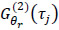, for different values of the time delay τj. In multi-angle DLS (MDLS), several measurements are taken at different θr, where r = (1,2,…,R), and R is the number of measurement angles, and each of them is related to the (first-order and normalized) autocorrelation functions of the electric field,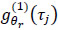through (1):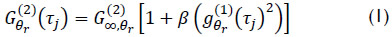where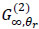is the measured baseline; β (<1) is an instrumental parameter; j = 1, 2, ..., M; and M is the number of points of the autocorrelation functions, limited by the number of available correlator channels.

Call f(Di) the discrete number PSD, where each ordinate of f(Di) represents the number-fraction of particles contained in the diameter interval [Di, Di+1] (i = 1, 2, …, N). All the Di values are evenly-spaced at diameter intervals ΔD along the diameter range [Dmin, Dmax]. For a given angle,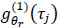is related to f(Di) as follows (Gugliotta et al., 2000; Vega et al., 2003) through (2):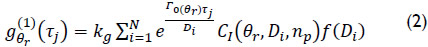where kg are the (a priori unknown) proportionality constants that adopt different values at each θr; CI is the fraction of light intensity scattered by a particle of diameter Di and refractive index np, at the angle θr, for fixed values of the laser light polarization and the laser wavelength. Coefficients CI can be calculated through the Mie scattering theory (Bohren et al., 1983), and Γ0(θr) is defined through (3):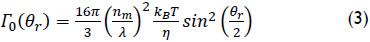where λ is the in-vacuum wavelength of the incident laser light; nm is the refractive index of the non-absorbing medium; kB (= 1.38×10-23 Kg.m2.s-2.K-1) is the Boltzmann constant; T is the absolute temperature; and η is the medium viscosity at T.

By inverting (2) it is possible to estimate the PSD on the basis of either a single-angle DLS measurement (Gugliotta et al., 2000), or multi-angle DLS (MDLS) measurements (Vega et al., 2003). The estimation procedure requires solving a linear ICIP through an appropriate regularization scheme. An alternative approach has been proposed in order to improve the conditioning of the inverse problem. It consists of replacing (2) by an expression representing different average diameters of the PSD, which are directly calculated from the DLS measurements at each ϑr. Such diameters [that we shall call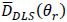] can accurately be calculated from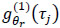by means of the well-known method of cumulants (Koppel, 1972). Most commercial DLS equipment is equipped with software that evaluates. The nonlinear relationship betweenand f(Di) is given by (4) (Bermeo et al., 2010; Clementi et al., 2011, 2012a, 2012b, Gugliotta et al., 2009; Vega et al., 2003):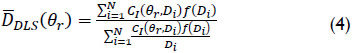To estimate the PSD from (4) on the basis of, a non-linear ICIP must be solved.

A classical approach for solving both linear and non-linear ICIP is the constraint Tikhonov regularization method (Tikhonov et al., 1977). On the basis of (4), such method can be seen as an optimization problem of the form (5):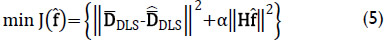with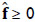, where α is the regularization parameter, which can automatically be determined through the L-curve method (Farquharson et al., 2004); H (N×N) is the regularization matrix (typically, the discrete second derivative operator);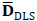(R×1) is the vector whose components are the measured average diametersr), as determined by the method of cumulants (Koppel, 1972);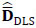(R×1) contains the average diametersr) obtained through (4) for the estimated PSD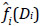; and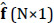contains the discrete heights of the estimated PSD. Due to the ill-conditioning of the inverse problem, (5) has several local minima; and for this reason, the utilization of an efficient global optimization technique is required.

Particle Swarm Optimization

PSO proved to be a powerful tool for solving linear and nonlinear optimization problems in continuous and discrete spaces. In a PSO system, the search is performed using particle populations. Each particle corresponds to an individual, which represents a candidate solution to the considered problem. The particles change their state by “flying” through the search space until some relatively stable state is reached (Kennedy et al., 1995, 1997, 2001; Ozcan et al., 1999; Rocca et al., 2009).

A PSO system combines an “exclusively social model” (which suggests that individuals ignore their own experience and adjust their knowledge according to the success of individuals in the neighborhood), with an “exclusively cognitive model” (which treats the individuals as isolated beings). A particle changes its position using these two conceptual models.

In a PSO algorithm, the i-th particle is treated as a point within a space of N dimensions, and it is represented by a vector: Xi = (xi, 1, ..., xi, N). The best position found by the i-th particle, i.e. the position that produced the best value of the objective function (5), is represented by Pi = (pi, 1, ..., pi, N); and the best position found by the entire population is represented by G = (g1, ..., gN). The velocity for the i-th particle is represented by Vi = (vi, 1, ..., vi, N). At each iteration t, the particles are manipulated according to the following model, through (6,7) (Kennedy et al., 2001):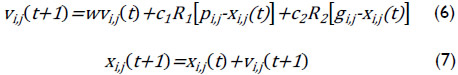where c1 and c2 are the so-called ‘cognitive’ and ‘social’ accelerations, respectively; R1 and R2 are two random numbers in the range 0 - 1; and w is the inertia weight (Rocca et al., 2009). Equation (6) is used to calculate the new particle velocity according to its previous velocity {vi,j(t)}, and to its current location distance {xi,j(t)} to its best position {pi,j}, and to the best position within the population {gj}. Then, the particle moves to a new position according to (7). The inertia weight, w, is used to control the previous velocity impact on the current velocity, thus influencing the exploration skills of a particle at the global (wide) or local (short) range. A larger inertia weight facilitates global exploration to find new areas, while a smaller inertia weight tends to facilitate local exploration to reach a finer tune on the current search area. Proper selection of cognitive and social accelerations, as well as inertia weight, can provide a balance between the local and global abilities search and therefore a reduced number of iterations are required to find the optimum solution.

A pseudo-code for implementing the PSO algorithm is shown in Table 1.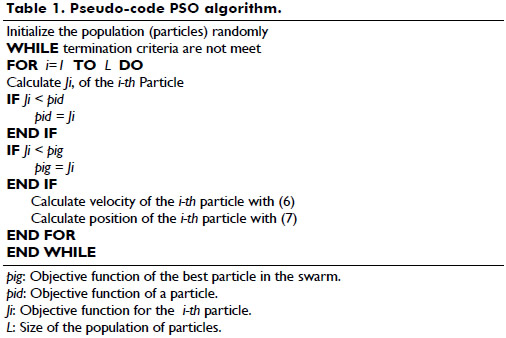Several authors had reviewed the basic concepts of PSO algorithms; and as a consequence, different variations of the canonical form were proposed. Some of the most relevant PSO algorithms are: 1) the linearly decreasing inertia weight PSO (LDWPSO), where the parameter w is usually variable in a range from 0.1 to 0.9 (Shi et al., 2001); 2) the PSO with Trelea’s first parameter set (T1PSO), and the PSO with Trelea’s second parameter set (T2PSO), where the parameters w, c1 and c2 are fixed on the basis of Trelea (2003); and 3) the constriction factor PSO (CFPSO), where velocity is modified by the so-called constriction factor k, the inertia weight is suppressed, and (6) is replaced by (8) (Clerc, 1999):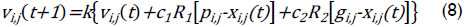Test with Simulated Examples

To evaluate the performance of the PSOs, three PSDs corresponding to hypothetical polystyrene (PS) latex with particles in the diameter range [100 nm, 500 nm] were considered. In all cases, the discrete diameter axis was evenly-spaced at fixed intervals of ΔD = 10 nm, and therefore the PSD is described by N = 41 points.

The first PSD, f1(Di), was chosen as a narrow and asymmetric log-normal distribution, with a geometric average diameter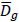= 203 nm, and a standard deviation σL = 0.115 nm; through (9).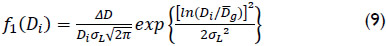The second PSD, f2(Di) was chosen as an asymmetric EMG distribution, which was obtained by convoluting a Gaussian [of mean diameter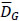= 225 nm, and standard deviation σG = 35 nm, with a decreasing exponential function of decay constant τ = 20 nm; though (10).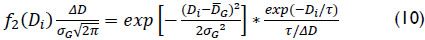where the symbol ‘*’ represents the convolution product.

The third PSD, f3(Di), was chosen as a bimodal distribution obtained by combining two log-normal distributions, f3,1(Di) and f3,2(Di), with relative amounts of particles 85% and 15%, respectively; though (11).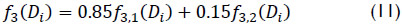where f3,1(Di) and f3,2(Di) are calculated from (9), with the following parameters for each mode: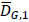= 250 nm, σL,1 = 0.115 nm, for f3,1, and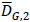= 400 nm, σL,2 = 0.05 nm, for f3,2.

For simulating the MDLS measurements a wavelength λ = 632.8 nm vertically-polarized He-Ne laser was assumed. At this laser wavelength, the refractive indexes are: np = 1.5729, for the PS particles, and nm = 1.3319, for the dispersion medium (pure water). The measurements were simulated at a constant temperature of 30°C (T = 303.15 K), and therefore η = 0.798 g/(m.s). The measurements angles ranged from 30º to 140º, at regular intervals of 10º, for the examples. These parameters were used to evaluate CI(qr, Di, np) through the Mie theory, and are required to simulate the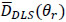measurements through (1-3), together with the method of cumulants. To reach representative simulations of the real cases, a numerical noise was added to each simulated measurement. To simulate the noisy autocorrelation measurements,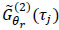, the noise-free autocorrelations,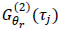, obtained through (1-3) were contaminated with additive random noises of a similar magnitude to those observed in the experiments, as follows: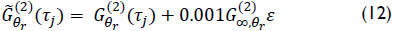where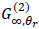is the measured baseline (obtained as described in [Vega, et al., 2003]); and ε is a Gaussian random sequence of mean zero and variance one. From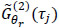, the noisy first-order autocorrelation function of the electric field,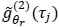was calculated through (1); and the noisy measurements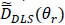were obtained through the method of cumulants, Figs. 1.b), 2.b), and 3.b). These values were fed into the proposed PSOs for estimating the simulated PSDs f1, f2 and f3, thus yielding the estimates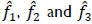, respectively, as shown in Figs. 1.a), 2.a) and 3.a).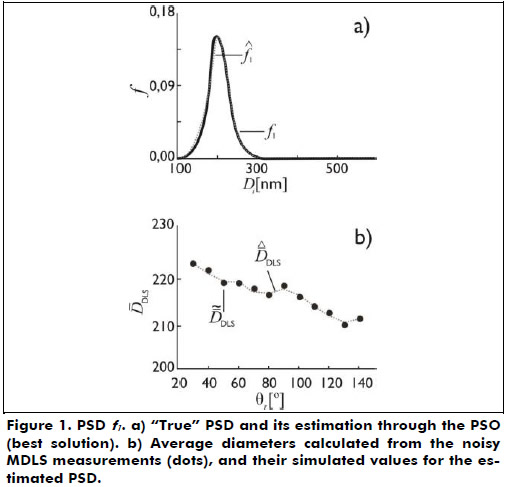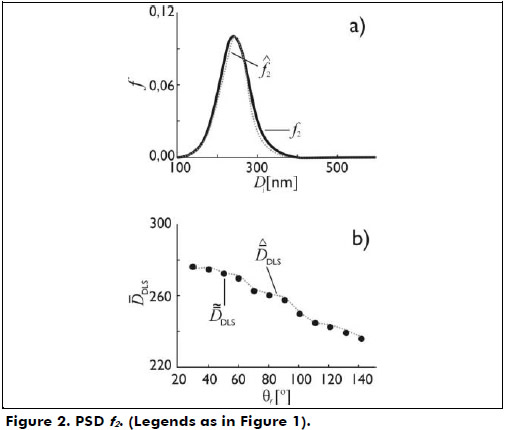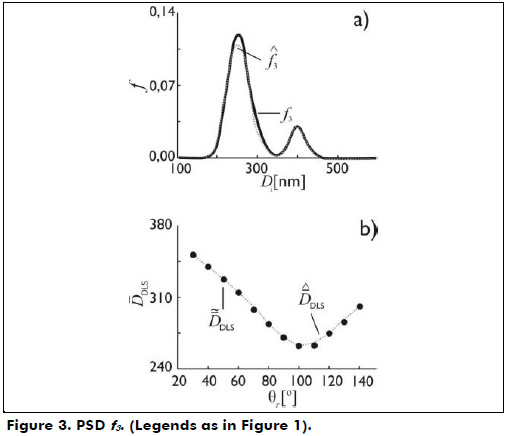Five different PSO schemes were implemented to solve the ICIP of (5). Table 2 shows the implemented PSOs, together with their functional parameters, c1, c2, w, and k (Kennedy et al., 2001; Shi et al., 1998; Trelea, 2003). The variable wl correspond to a linearly decreasing inertia function that starts at a value of wl = 0.9 for the first iteration, and then linearly decreases to wl = 0.1, for the last iteration.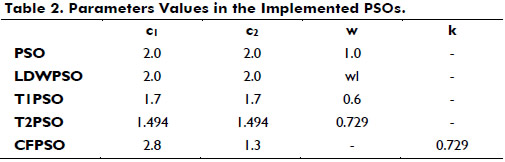In all the implemented PSO, 50 particles were chosen. These values are similar to the number of variables that must be estimated. The stop criterion is adopted when the objective function (5) reaches the value 1×10-10, or when the number of iterations reaches 15000.

All the analyzed cases were solved with each implemented PSO, Table 3 shows the minimum value of the objective function (5) (Jmin), the average value (Javg) and the standard deviation (σ) of the objective function values for the entire final population. It can be seen that T1PSO showed the best performance, as indicated for the smallest Jmin.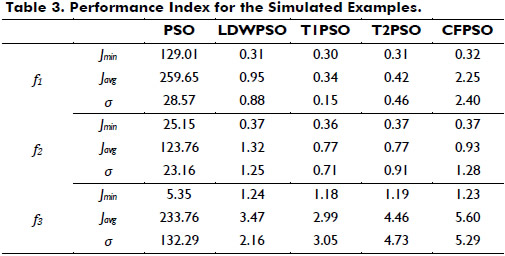Table 3 presents the values of the objective function obtained for the best particle of each simulated method. In all cases, T1PSO has shown a fast improvement in the value of the objective function in comparison with the others PSOs implemented.

Figures 1.a), 2.a), and 3.a) show the estimated PSDs obtained by T1PSO, that produces in all cases the smallest Jmin. The estimated PSDs adequately reproduce the simulated distributions. The estimated average diameters,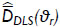, can be obtained by introducing the estimated PSD into (4). From Figs. 1.b), 2.b), and 3.b), the retrievedalmost exactly reproduces the noisy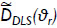.

Test with Experimental Examples

The method was experimentally tested on the basis of 2 PS latexes with bimodal PSDs, obtained by simply mixing 2 PS standards (from Polysciences) of 306 nm and 974 nm nominal diameters. The number-fraction of the larger-sized particles was gravimetrically determined, for the first latex f4 the proportion are: ≈ 98.95% and ≈ 1.05%, and for the second latex f5 the number-fraction of the larger-sized particles are: ≈ 97.90% and ≈ 2.10%. These experimental cases are difficult to be solved because they involve the estimation of 2 narrow peaks of quite different magnitudes and placed in a wide range of possible diameters. It is important to check the capability of the proposed algorithm, in order to successfully estimate the nominal diameter of both modes and their number-fractions.

MDLS measurements were acquired with a general purpose laser light-scattering photometer (Brookhaven Instruments, Inc.) fitted with a vertically-polarized He–Ne laser (λ = 632.8 nm), and a digital correlator (model BI-2000 AT). The measurements were carried out at 30 °C (T = 303.15 K). The total measurement times ranged from 200 s to 500 s. The measurements angles ranged from 30º to 140º, and in both experimental examples the simulations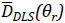were calculated from the measured autocorrelation functions through the quadratic method of cumulants. Both examples were previously characterized by transmission electron microscopy (TEM).

Table 4 presents the values of the objective function obtained by the best particle of each implemented method. In the first case, T1PSO has shown a fast improvement in the value of the objective function in comparison with the others implemented PSOs. In the second case, CFPSO provides the minimum of the objective function in comparison with the others implemented methods. Note that, in general, T1PSO and CFPSO also produced the best estimates of the average diameters for the first and second experimental cases, respectively. These results suggest that the population of particles is close to the problem solution.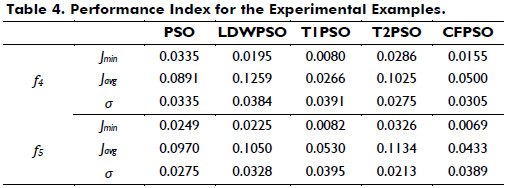Figures 4 and 5 show the estimated PSDs obtained by T1PSO and CFPSO respectively, that produces in both cases the smallest Jmin. The estimated PSDs and average diameters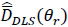, adequately reproduce the experimental examples.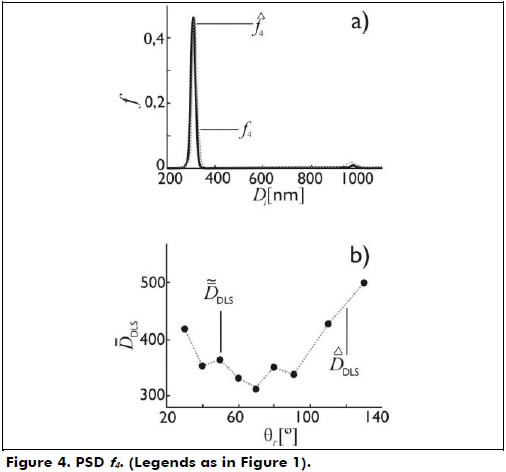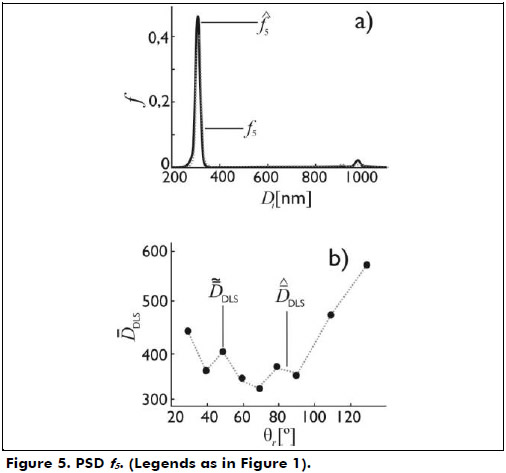Conclusions

Several PSOs were implemented for estimating the particle size distribution of polymeric latexes from multi-angle dynamic light scattering measurements. The estimation procedure was based on the minimization of a functional according to the Tikhonov regularization method. The PSOs were tested on the basis of simulated and experimental examples involving unimodal and bimodal PSDs, with different shapes and widths. The simulation results showed that the PSOs are robust algorithms for estimating PSDs from MDLS measurements. In all of the analyzed examples, the PSO with Trelea’s parameter set showed better performance, in the sense that it produced a smallest J, and an adequate average value of J when compared with other implemented PSOs.

Acknowledgment

Univ. del Valle (Colombia); Univ. Nac. del Litoral, CONICET and UTN-FRSF (Argentina); Univ. Federal de Rio de Janeiro, FAPERJ, CAPES and CNPq (Brazil).

References

Barandiaran, M., de la Cal, J. C., & Asua, J. M. (2007). Emulsion polymerization. Polymer reaction engineering. Oxford: J. M. Asua, Blackwell Pub.         [ Links ]

Bermeo, L. A., Clementi, L. A, Caicedo, E., Vega, J. R., & Muñoz, M. (2010). Sizing nanoparticles from dynamic light scattering measurements: II. Evaluation of particle swarm optimization strategies. Inverse Problem, Design and optimization Symposium, IPDO2010. Joao Pessoa, Brazil.         [ Links ]

Bohren, C., Huffman, D. (1983). Absorption and scattering of light by small particles. New York: J. Wiley & Sons.         [ Links ]

Clementi, L. A., Orlande, H. R. B., Gugliotta, L. M., & Vega, J. R. (2011). A Bayesian inversion method for estimating the particle size distribution of latexes from multiangle dynamic light scattering measurements. Chemometrics and Intelligent Laboratory Systems, 107(1), 165-173.         [ Links ]

Clementi, L. A., Vega, J. R., Gugliotta, L. M. (2012). Particle size distribution of multimodal polymer dispersions by multiangle dynamic light scattering. Solution of the inverse problem on the basis of a genetic algorithm. Part. & Part. Syst. Charact., 27(5-6), 146-157.         [ Links ]

Clementi, L. A., Vega, J. R., Orlande, H. R. B., & Gugliotta, L. M. (2012) Sizing nanoparticles from Dynamic Light Scattering. Comparison of Bayesian and Tikhonov inversion methods. Inverse Problem in Sciencies and Engineering, 20(7), 973-990.         [ Links ]

Clerc, M. (1999). The swarm and the queen: Towards a deterministic and adaptive particle swarm optimization. 1999 Congress on Evolutionary Computation-CEC 99. Washington, DC, USA.         [ Links ]

Farquharson, C. G., & Oldenburg, D. W. (2004). A comparison of automatic techniques for estimating the regularization parameter in non-linear inverse problems. Geophys. J. Int., 156(3), 411-425.         [ Links ]

Gilbert, R. G. (1995). Emulsion Polymerization. A Mechanistic Approach. London: Academic Press.         [ Links ]

Gugliotta, L. M., Stegmayer, G. S., Clementi, L. A., Gonzalez, V. D. G., Minari, R. J., Leiza, J. R., & Vega, J. R. (2009). A neural network model for estimating the particle size distribution of a dilute latex from multiangle dynamic light scattering measurements. Part. & Part. Syst. Charact., 26(1-2), 41-52.         [ Links ]

Gugliotta, L. M., Vega, J. R., & Meira, G. R. (2000). Latex particle size distribution by dynamic light scattering: Computer evaluation of two alternative calculation paths. J. of Col. and Int. Sci., 228(1), 14-17.         [ Links ]

Kennedy, J., & Eberhart, R. (1995). Particle swarm optimization. In Proceedings of IEEE International Conference on Neural Networks (pp. 1942-1948).         [ Links ]

Kennedy, J., & Eberhart, R. (1997). A discrete binary version of the particle swarm algorithm. In Proceedings of the World Multiconference on Systemics, Cybernetics and Informatics (pp. 4104-4109).         [ Links ]

Kennedy, J., & Eberhart, R. (2001). Swarm intelligence. United State of America: Academic Press.         [ Links ]

Kirsch, A. (1996). An Introduction to the Mathematical Problem of Inverse Problems. New York: Springer-Verlag.         [ Links ]

Koppel, D. E. (1972). Analysis of macromolecular polydispersity in intensity correlation spectroscopy: The method of cumulants. J. Chem. Phys., 57, 4814-4820.         [ Links ]

Liu, X., Shen, J., Thomas, J. C., Clementi, L. A., & Sun, X. (2012). Multiangle dynamic light scattering analysis using a modified Chahine method. Journal of Quantitative Spectroscopy and Radiative Transfer, 113(6), 489-497.         [ Links ]

Ozcan, E., & Mohan, C. K. (1999). Particle swarm optimization: Surfing the waves. In Memories of IEEE Congress on Evolutionary Computation-CEC99. Washington, DC, USA.         [ Links ]

Rocca, P., Benedetti, M., Donelli, M., Franceschini, D., & Massa, A. (2009). Evolutionary optimization as applied to inverse scattering problems. Inverse Problems, 25(12), 1-41.         [ Links ]

Shi, Y., & Eberhart, R. (1998). Parameter selection in particle swarm optimization. Evolutionary Programming VII Lecture Notes in Computer Science, 1447, 591-600.         [ Links ]

Tikhonov, A., & Arsenin, V. (1977). Solutions of illposed problems. Washington: Wiley.         [ Links ]

Trelea, I. C. (2003). The particle swarm optimization algorithm: Convergence analysis and parameter selection. Information Processing Letters, 85, 317-325.         [ Links ]

Vega, J. R., Gugliotta, L. M., Gonzalez, V. D. G., & Meira, G. R. (2003). Latex Particle Size Distribution by Dynamic Light Scattering. A Novel Data Processing for Multi-angle Measurements. J. of Col. and Int. Sci., 261(1), 74-81.         [ Links ]